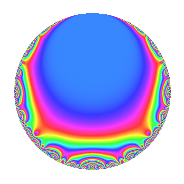# Properties

 Label 945.2.a.kLevel 945 Weight 2 Character orbit 945.a Self dual Yes Analytic conductor 7.546 Analytic rank 0 Dimension 2 CM No Inner twists 1

# Related objects

## Newspace parameters

 Level: $$N$$ = $$945 = 3^{3} \cdot 5 \cdot 7$$ Weight: $$k$$ = $$2$$ Character orbit: $$[\chi]$$ = 945.a (trivial)

## Newform invariants

 Self dual: Yes Analytic conductor: $$7.54586299101$$ Analytic rank: $$0$$ Dimension: $$2$$ Coefficient field: $$\Q(\sqrt{2})$$ Coefficient ring: $$\Z[a_1, a_2]$$ Coefficient ring index: $$1$$ Fricke sign: $$-1$$ Sato-Tate group: $\mathrm{SU}(2)$

## $q$-expansion

Coefficients of the $$q$$-expansion are expressed in terms of $$\beta = \sqrt{2}$$. We also show the integral $$q$$-expansion of the trace form.

 $$f(q)$$ $$=$$ $$q + ( 1 + \beta ) q^{2} + ( 1 + 2 \beta ) q^{4} + q^{5} - q^{7} + ( 3 + \beta ) q^{8} +O(q^{10})$$ $$q + ( 1 + \beta ) q^{2} + ( 1 + 2 \beta ) q^{4} + q^{5} - q^{7} + ( 3 + \beta ) q^{8} + ( 1 + \beta ) q^{10} + ( -1 + \beta ) q^{11} + ( 1 + \beta ) q^{13} + ( -1 - \beta ) q^{14} + 3 q^{16} + ( 2 + 2 \beta ) q^{17} + ( 3 - \beta ) q^{19} + ( 1 + 2 \beta ) q^{20} + q^{22} + ( 2 - 2 \beta ) q^{23} + q^{25} + ( 3 + 2 \beta ) q^{26} + ( -1 - 2 \beta ) q^{28} -4 q^{29} + 6 q^{31} + ( -3 + \beta ) q^{32} + ( 6 + 4 \beta ) q^{34} - q^{35} -6 \beta q^{37} + ( 1 + 2 \beta ) q^{38} + ( 3 + \beta ) q^{40} + ( -5 - 2 \beta ) q^{41} + ( -1 - 4 \beta ) q^{43} + ( 3 - \beta ) q^{44} -2 q^{46} + ( -1 + 6 \beta ) q^{47} + q^{49} + ( 1 + \beta ) q^{50} + ( 5 + 3 \beta ) q^{52} + ( -1 - 7 \beta ) q^{53} + ( -1 + \beta ) q^{55} + ( -3 - \beta ) q^{56} + ( -4 - 4 \beta ) q^{58} + ( 2 - 6 \beta ) q^{59} -6 \beta q^{61} + ( 6 + 6 \beta ) q^{62} + ( -7 - 2 \beta ) q^{64} + ( 1 + \beta ) q^{65} + ( 5 + 2 \beta ) q^{67} + ( 10 + 6 \beta ) q^{68} + ( -1 - \beta ) q^{70} + 2 q^{71} + ( 5 - 5 \beta ) q^{73} + ( -12 - 6 \beta ) q^{74} + ( -1 + 5 \beta ) q^{76} + ( 1 - \beta ) q^{77} + ( 12 + 2 \beta ) q^{79} + 3 q^{80} + ( -9 - 7 \beta ) q^{82} + ( 1 - 6 \beta ) q^{83} + ( 2 + 2 \beta ) q^{85} + ( -9 - 5 \beta ) q^{86} + ( -1 + 2 \beta ) q^{88} + ( -3 - 4 \beta ) q^{89} + ( -1 - \beta ) q^{91} + ( -6 + 2 \beta ) q^{92} + ( 11 + 5 \beta ) q^{94} + ( 3 - \beta ) q^{95} + 10 \beta q^{97} + ( 1 + \beta ) q^{98} +O(q^{100})$$ $$\operatorname{Tr}(f)(q)$$ $$=$$ $$2q + 2q^{2} + 2q^{4} + 2q^{5} - 2q^{7} + 6q^{8} + O(q^{10})$$ $$2q + 2q^{2} + 2q^{4} + 2q^{5} - 2q^{7} + 6q^{8} + 2q^{10} - 2q^{11} + 2q^{13} - 2q^{14} + 6q^{16} + 4q^{17} + 6q^{19} + 2q^{20} + 2q^{22} + 4q^{23} + 2q^{25} + 6q^{26} - 2q^{28} - 8q^{29} + 12q^{31} - 6q^{32} + 12q^{34} - 2q^{35} + 2q^{38} + 6q^{40} - 10q^{41} - 2q^{43} + 6q^{44} - 4q^{46} - 2q^{47} + 2q^{49} + 2q^{50} + 10q^{52} - 2q^{53} - 2q^{55} - 6q^{56} - 8q^{58} + 4q^{59} + 12q^{62} - 14q^{64} + 2q^{65} + 10q^{67} + 20q^{68} - 2q^{70} + 4q^{71} + 10q^{73} - 24q^{74} - 2q^{76} + 2q^{77} + 24q^{79} + 6q^{80} - 18q^{82} + 2q^{83} + 4q^{85} - 18q^{86} - 2q^{88} - 6q^{89} - 2q^{91} - 12q^{92} + 22q^{94} + 6q^{95} + 2q^{98} + O(q^{100})$$

## Embeddings

For each embedding $$\iota_m$$ of the coefficient field, the values $$\iota_m(a_n)$$ are shown below.

For more information on an embedded modular form you can click on its label.

Label $$\iota_m(\nu)$$ $$a_{2}$$ $$a_{3}$$ $$a_{4}$$ $$a_{5}$$ $$a_{6}$$ $$a_{7}$$ $$a_{8}$$ $$a_{9}$$ $$a_{10}$$
1.1
 −1.41421 1.41421
−0.414214 0 −1.82843 1.00000 0 −1.00000 1.58579 0 −0.414214
1.2 2.41421 0 3.82843 1.00000 0 −1.00000 4.41421 0 2.41421
 $$n$$: e.g. 2-40 or 990-1000 Significant digits: Format: Complex embeddings Normalized embeddings Satake parameters Satake angles

## Inner twists

This newform does not admit any (nontrivial) inner twists.

## Atkin-Lehner signs

$$p$$ Sign
$$3$$ $$1$$
$$5$$ $$-1$$
$$7$$ $$1$$

## Hecke kernels

This newform can be constructed as the intersection of the kernels of the following linear operators acting on $$S_{2}^{\mathrm{new}}(\Gamma_0(945))$$:

 $$T_{2}^{2} - 2 T_{2} - 1$$ $$T_{11}^{2} + 2 T_{11} - 1$$